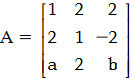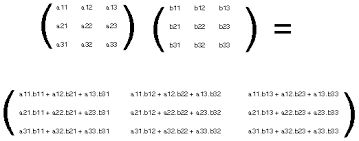# ifis a matrix satisfying the equation AAT=9I, where I is 3x3 identity matrix, then the ordered pair(a, b) is equal to : Option 1)Option 2)Option 3)Option 4)Using

Multiplication of matrices --

by given condition

Option 1)This option is incorrect.

Option 2)This option is incorrect.

Option 3)This option is incorrect.

Option 4)This option is correct.

Exams
Articles
Questions